# GED Math : Single-Variable Algebra

## Example Questions

### Example Question #81 : Solving For The Variable

Solve: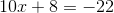Possible Answers: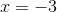Correct answer:Explanation:

Subtract the 8 from the left and add (subtract) from the right: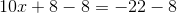Simplify: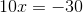Divide by 10: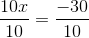Simplify:### Example Question #687 : Ged Math

Solve: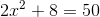Possible Answers: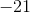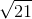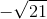Correct answer:Explanation:

Step 1: Move the constant from the left to the right: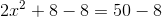Step 2: Simplify: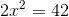Step 3: Divide by the coefficient on the left hand side to both sides of the equation: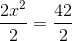Simplify: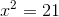Step 4: Take the square root of both sides: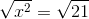Simplify: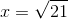### Example Question #1 : Word Problems In Algebra

Which of the following phrases can be written as the algebraic expression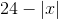?

Possible Answers:

The opposite of the difference of a number and twenty-four

Twenty-four decreased by the absolute value of a number

Twenty-four decreased by the opposite of a number

The absolute value of a number decreased by twenty-four

Correct answer:

Twenty-four decreased by the absolute value of a number

Explanation:is twenty-four decreased by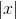, which is the absolute value of a number, sois "twenty-four decreased by the absolute value of a number."

### Example Question #1 : Word Problems In Algebra

Which of the following phrases can be written as the algebraic expression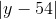?

Possible Answers:

The opposite of the product of a number and negative fifty-four

The absolute value of the difference of a number and fifty-four

The absolute value of the product of a number and negative fifty-four

The opposite of the difference of a number and fifty-four

Correct answer:

The absolute value of the difference of a number and fifty-four

Explanation:is the absolute value of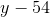, which is the difference of a number and fifty-four; therefore,is "the absolute value of the difference of a number and fifty-four."

### Example Question #3 : Word Problems In Algebra

Which of the following phrases can be written as the algebraic expression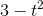?

Possible Answers:

Three decreased by the square of a number

The square of the difference of three and a number

The square of the difference of a number and three

The square of a number decreased by three

Correct answer:

Three decreased by the square of a number

Explanation:is three decreased by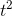, which is the square of a number; therefore,is three decreased by the square of a number.

### Example Question #1 : Word Problems In Algebra

Which of the following phrases can be written as the algebraic expression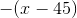?

Possible Answers:

The opposite of the difference of a number and forty-five

The opposite of the difference of forty-five and a number

The absolute value of the difference of forty-five and a number

The absolute value of the difference of a number and forty-five

Correct answer:

The opposite of the difference of a number and forty-five

Explanation:is the opposite of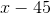, which is the difference of a number and forty-five; therefore,is the opposite of the difference of a number and forty-five.

### Example Question #1 : Word Problems In Algebra

Which of the following phrases can be written as the algebraic expression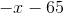?

Possible Answers:

The absolute value of the difference of a number and sixty-five

Sixty-five subtracted from the opposite of a number

Sixty-five subtracted from the absolute value of a number

The opposite of the difference of a number and sixty-five

Correct answer:

Sixty-five subtracted from the opposite of a number

Explanation:is sixty-five subtracted from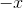, which is the opposite of a number; therefore,is "sixty-five subtracted from the opposite of a number."

### Example Question #6 : Word Problems In Algebra

Which of the following phrases can be written as the algebraic expression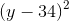?

Possible Answers:

The square of the difference of thirty-four and a number

Twice the difference of a number and thirty-four

The square of the difference of a number and thirty-four

Twice the difference of thirty-four and a number

Correct answer:

The square of the difference of a number and thirty-four

Explanation:is the square of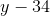, which is the difference of a number and thirty-four. Therefore,is the difference of a number and thirty-four.

### Example Question #1 : Word Problems In Algebra

A broken television cost $1.25 after a 99% discount. What was the original price? Possible Answers:$2.49

$250$125

$2.74$12.50

Correct answer:

\$125

Explanation:

Let x be the original price.  If the original price was given a discount, the value of the percent discount must be subtracted from the original price.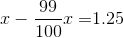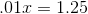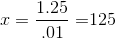### Example Question #8 : Word Problems In Algebra

Which of the following phrases can be represented by the algebraic expression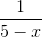?

Possible Answers:

Five decreased by the reciprocal of a number

Five less than by the reciprocal of a number

The reciprocal of the difference of a number and five

The reciprocal of the difference of five and a number

Correct answer:

The reciprocal of the difference of five and a number

Explanation:is the reciprocal of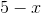, which is the difference of five and a number. Therefore,is "the reciprocal of the difference of five and a number".

### All GED Math Resources